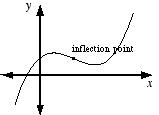index: click on a letter A B C D E F G H I J K L M N O P Q R S T U V W X Y Z A to Z index index: subject areas numbers & symbols sets, logic, proofs geometry algebra trigonometry advanced algebra & pre-calculus calculus advanced topics probability & statistics real world applications multimedia entrieswww.mathwords.com about mathwords website feedback

 Inflection Point A point at which a curve changes from concave up to concave down, or vice-versa. Note: If a function has a second derivative, the value of the second derivative is either 0 or undefined at each of that function's inflection points.See also